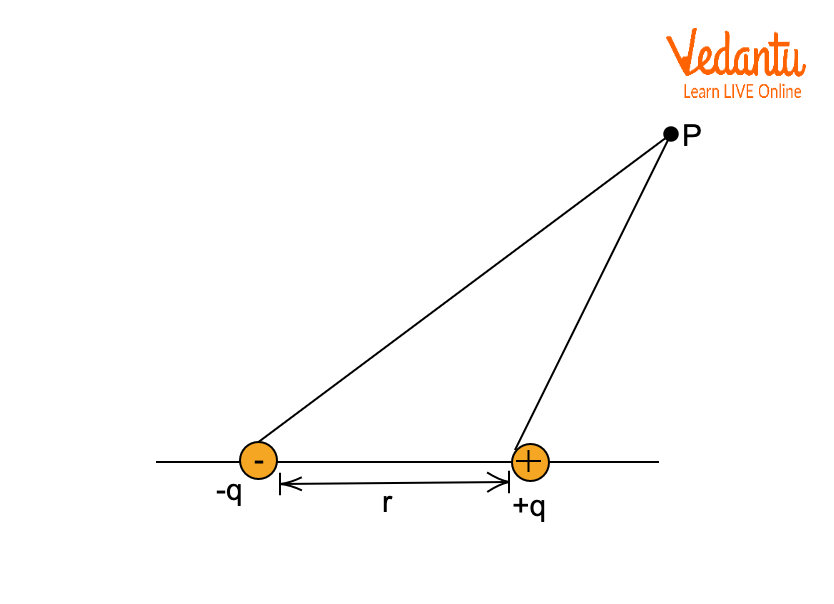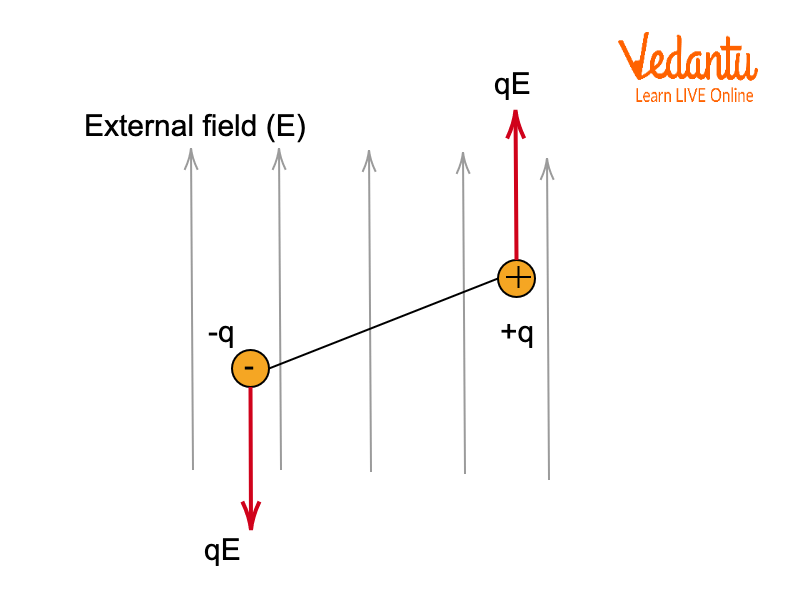Courses
Courses for Kids
Free study material
Free LIVE classes
More

# Torque on an Electric Dipole Placed in a Uniform Electric Field for JEE## What is an Electric Dipole?

Last updated date: 27th Mar 2023
Total views: 70.8k
Views today: 0.48k

Changes come in a various assortment of forms, and when present in a stimulating field, they can show a range of characteristics varying in different conditions. These charges are the main elements of creating an electric dipole. An electric dipole, to be more precise, is the division of positive and negative charges by a distance. Every electric dipole experiences torque as they produce an electric field.

An electric dipole is a pair of opposite electric charges with equal magnitudes that are spaced apart by distance d. The product of the size of these charges and their separation from one another is the electric dipole moment for this. In the dipole moment, a vector quantity can describe dipoles, whether they are electric or magnetic. For a simple electric dipole, it can be said that the electric dipole moment is a vector pointing from the negative charge to the positive charge, and its magnitude is equal to the sum of the charges' individual strengths times their distance from one another. The electric dipole moment formula is expressed by

$p=qd$

where the magnitude of the charge is q and d is the distance between the charges.

## Derivation of Electric Potential due to an Electric Dipole

Consider an electric dipole with given charges +q and –q on the X-axis originating from the midpoint of the dipole. Let's take point P at the coordinates (x,y). The angle that the positive x-axis makes with the point's distance from the origin, R.Electric Potential due to an Electric Dipole

$V=\frac{kq}{r}$  represents the electric potential caused by a charge q at a point that is separated from the charge by a distance of r. Hence, at point P, the potential for charge +q is V1, and for the charge –q is V2. Hence, the net electric potential due to an electric dipole is expressed with the equation,

$V=\frac{KP\cos \theta }{{{r}^{2}}-{{a}^{2}}{{\cos }^{2}}\theta }$

For a point on the dipole's equatorial axis, $\theta =\frac{\pi }{2}$;

$V=\frac{KP\cos \left( \frac{\pi }{2} \right)}{{{r}^{2}}-{{a}^{2}}{{\cos }^{2}}\left( \frac{\pi }{2} \right)}$

$V=\frac{KP\left( 0 \right)}{{{r}^{2}}-{{a}^{2}}\left( 0 \right)}$

$V =0$

Now that a dipole has been established, let's learn how to calculate torque.

## Torque Measurement

Torque can be defined as measuring the force that propels something around an axis. It is a vector quantity, and the force applied on the axis determines the direction of the Torque. The magnitude of the vector quantity is determined by

$\tau =Fr\sin \theta$

Here,

F is the force on the axis,

r is the moment arm length, and

$\theta$ is the angle produced by the force vector and moment arm.

## Torque on an Electric Dipole

Take a dipole in a uniform external field (E) in order to calculate the Torque that a dipole will experience when it lies on an external field. The electric force acting on the positive charge will be of 'qE' magnitude in the upward direction, whereas the electric force acting on the negative charge will be of 'qE' magnitude in the downward direction.Torque on an Electric Dipole

It is evident that the dipole is in translational equilibrium since the net force is zero. Let's know about rotational equilibrium here.  In this case, the dipole can stay stationary but rotate at a specific rate. Experiments have shown that both electrostatic forces (qE) function as clockwise torques. The dipole rotates as a result when exposed to a uniform external electric field.

Torque always functions with applied external force, which always occurs in pairs. Moreover, the strength and length of its arm contribute to its magnitude. The distance between the point of application of the force and the point at which the dipole rotates is known as the arm.

## Calculating Torque in a Uniform Electric Field

Consider a dipole where the charges +q and -q form a dipole, and they are separated by distance d. Place it in a uniform electric field with strength E so that the dipole's axis makes an angle with the electric field, θ.

Hence, the force is expressed when perpendicular to the dipole is,

$F_{+}^{\bot }=+q\sin \theta$

$F_{-}^{\bot }=-q\sin \theta$

Due to the equal force magnitudes and distance d, the Torque on the dipole is determined by,

Torque $\left( \tau \right)$ = Force X Distance Separating Forces

$\tau =dqE\sin \theta$

It is prior established that dipole moment p = qd

If the electric field is positive, the Torque is in a clockwise direction. So the value would be –ve.

Hence,

$\tau =-pE\sin \theta$

Or, it can be expressed as,

$\overrightarrow{\tau }=\overrightarrow{p}\times \overrightarrow{E}$

$\left| \overrightarrow{\tau } \right|=\left| \overrightarrow{p}\times \overrightarrow{E} \right|=pE\sin \theta$

The electric field can be dimensionally represented by $\left[{M^1} {L^1} {I^{-1}} {T^{-3}}\right]$. So, the dimension of electric field intensity is $\left[M~L~{T^{-3}}~{A^{-1}}\right]$.

## Conclusion

Now it is evaluated that Torque is the term describing the force that rotates an item around an axis. The Torque on the dipole results from the force's magnitude divided by d, the distance separating the force vectors. Hence, the Torque on an electric dipole in a uniform electric field is derived by the above equation.

## FAQs on Torque on an Electric Dipole Placed in a Uniform Electric Field for JEE

1. What happens when a dipole is placed in an unequal electric field?

When put in the same electrical circuit, each dipole charger gains power, and the dipole vector direction does not match the field direction. Both forces are the same size but move in different directions. These opposite and equal forces make up the pair. The dipole rotates and aligns in place when the pair apply Torque to it. When the dipole is positioned differently, Torque will result. After the dipole is aligned with the field's direction, the force acting on cases is different. The electric dipole consequently experiences Torque and force in an unequal location.

2. What angle produces the highest Torque for the dipole in the external electric field?

When it comes to Torque experienced by a dipole in an external electric field, it can be evaluated through the below equation,

$\tau =pE\sin \theta$

As per the trigonometric functions, The sine function shows the maximum value at an angle of 90°. The value of sin 90° is 1. Hence, it is evaluated that the dipole experiences the highest Torque when at an angle of 90° in the electric field. So, it can be stated that the dipole experiences maximum Torque as long as it is held perpendicular to the electric field.